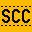# scc

simple c99 compiler
git clone git://git.simple-cc.org/scc

unpack.c (2889B)

```      1 #include <ctype.h>
2 #include <stdarg.h>
3 #include <stdio.h>
4 #include <stdlib.h>
5
6 #include <scc/mach.h>
7 #include "libmach.h"
8
9 static int
10 lunpack(unsigned char *src, char *fmt, va_list va)
11 {
12 	unsigned char *bp, *cp;
13 	unsigned short *sp;
14 	unsigned s;
15 	unsigned long *lp, l;
16 	unsigned long long *qp, q;
17 	int n;
18
19 	bp = src;
20 	while (*fmt) {
21 		switch (*fmt++) {
22 		case '\'':
23 			n = atoi(fmt);
24 			while (isdigit(*fmt))
25 				fmt++;
26 			cp = va_arg(va, unsigned char *);
27 			while (n--)
28 				*cp++ = *bp++;
29 			break;
30 		case 'c':
31 			cp = va_arg(va, unsigned char *);
32 			*cp = *bp++;
33 			break;
34 		case 's':
35 			sp = va_arg(va, unsigned short *);
36 			s =  (unsigned) *bp++;
37 			s |= (unsigned) *bp++ << 8;
38 			*sp = s;
39 			break;
40 		case 'l':
41 			lp = va_arg(va, unsigned long *);
42 			l = (unsigned long) *bp++;
43 			l |= (unsigned long) *bp++ << 8;
44 			l |= (unsigned long) *bp++ << 16;
45 			l |= (unsigned long) *bp++ << 24;
46 			*lp = l;
47 			break;
48 		case 'q':
49 			qp = va_arg(va, unsigned long long *);
50 			q = (unsigned long long) *bp++;
51 			q |= (unsigned long long) *bp++ << 8;
52 			q |= (unsigned long long) *bp++ << 16;
53 			q |= (unsigned long long) *bp++ << 24;
54 			q |= (unsigned long long) *bp++ << 32;
55 			q |= (unsigned long long) *bp++ << 40;
56 			q |= (unsigned long long) *bp++ << 48;
57 			q |= (unsigned long long) *bp++ << 56;
58 			*qp = q;
59 			break;
60 		default:
61 			return -1;
62 		}
63 	}
64
65 	return bp - src;
66 }
67
68 static int
69 bunpack(unsigned char *src, char *fmt, va_list va)
70 {
71 	unsigned char *bp, *cp;
72 	unsigned short *sp;
73 	unsigned s;
74 	unsigned long *lp, l;
75 	unsigned long long *qp, q;
76 	int n;
77
78 	bp = src;
79 	while (*fmt) {
80 		switch (*fmt++) {
81 		case '\'':
82 			n = atoi(fmt);
83 			while (isdigit(*fmt))
84 				fmt++;
85 			cp = va_arg(va, unsigned char *);
86 			while (n--)
87 				*cp++ = *bp++;
88 			break;
89 		case 'c':
90 			cp = va_arg(va, unsigned char *);
91 			*cp = *bp++;
92 			break;
93 		case 's':
94 			sp = va_arg(va, unsigned short *);
95 			s =  (unsigned) *bp++ << 8;
96 			s |= (unsigned) *bp++;
97 			*sp = s;
98 			break;
99 		case 'l':
100 			lp = va_arg(va, unsigned long *);
101 			l =  (unsigned long) *bp++ << 24;
102 			l |= (unsigned long) *bp++ << 16;
103 			l |= (unsigned long) *bp++ << 8;
104 			l |= (unsigned long) *bp++;
105 			*lp = l;
106 			break;
107 		case 'q':
108 			qp = va_arg(va, unsigned long long *);
109 			q =  (unsigned long long) *bp++ << 56;
110 			q |= (unsigned long long) *bp++ << 48;
111 			q |= (unsigned long long) *bp++ << 40;
112 			q |= (unsigned long long) *bp++ << 32;
113 			q |= (unsigned long long) *bp++ << 24;
114 			q |= (unsigned long long) *bp++ << 16;
115 			q |= (unsigned long long) *bp++ << 8;
116 			q |= (unsigned long long) *bp++;
117 			*qp = q;
118 			break;
119 		default:
120 			return -1;
121 		}
122 	}
123
124 	return bp - src;
125 }
126
127 int
128 unpack(int order, unsigned char *src, char *fmt, ...)
129 {
130 	int r;
131 	int (*fn)(unsigned char *dst, char *fmt, va_list va);
132 	va_list va;
133
134 	va_start(va, fmt);
135 	fn = (order == LITTLE_ENDIAN) ? lunpack : bunpack;
136 	r = (*fn)(src, fmt, va);
137 	va_end(va);
138
139 	return r;
140 }
```